Answer all questions and submit them either as an IPython notebook, LaTeX document, or Markdown document. Each question is worth 25 points.

## Question 1¶

The data below provides counts of a flour beetle (Tribolium confusum) population at various points in time:

In :
%matplotlib inline
import matplotlib.pyplot as plt
import numpy as np

days = 0,8,28,41,63,79,97,117,135,154
beetles = 2,47,192,256,768,896,1120,896,1184,1024

plt.plot(days, beetles)

Out:
[<matplotlib.lines.Line2D at 0x112dca150>]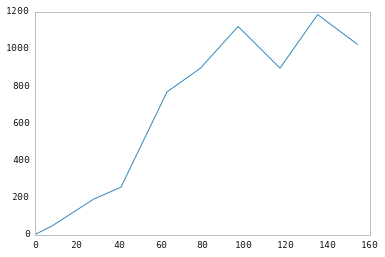An elementary model for population growth is the logistic model:

$$\frac{dN}{dt} = rN\left(1 - \frac{N}{K}\right)$$

where $N$ is population size, $t$ is time, $r$ is a growth rate parameter, and $K$ is a parameter that represents the population carrying capacity of the environment. The solution to this differential equation is given by:

$$N_t = f(t) = \frac{KN_0}{N_0 + (K - N_0)\exp(-rt)}$$

where $N_t$ denotes the population size at time $t$.

1. Fit the logistic growth model to the flour beetle data using the Newton–Raphson approach to minimize the sum of squared errors between model predictions and observed counts.

2. In many population modeling applications, an assumption of lognormality is adopted. The simplest assumption would be that the $\log(N_t)$ are independent and normally distributed with mean $\log[f(t)]$ and variance $\sigma^2$. Find the MLEs under this assumption, and provide estimates of standard errors and correlation between them.

### Solution to part 1¶

Here's an arbitrary (non-optimal) solution, which we can use as a starting point to improve:

In :
K0, r0 = 1000, 0.1

logistic = lambda t, K, r, N0=1.: K*N0 / (N0 + (K-N0) * np.exp(-r*t))

In :
times = np.arange(160)
plt.plot(times, logistic(times, K0, r0))
plt.plot(days, beetles, 'r.')

Out:
[<matplotlib.lines.Line2D at 0x1114bbd10>]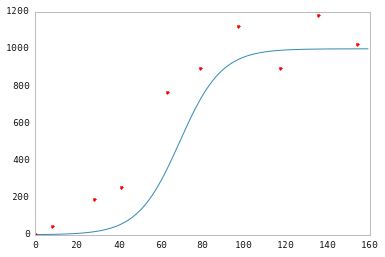In :
def mse(params):
K, r = params
return np.mean([(logistic(t, K, r) - y)**2 for t,y in zip(days, beetles)])

In :
mse([1000, 0.1])

Out:
34826.299294607059
In :
from scipy.optimize import fmin_l_bfgs_b

opt = fmin_l_bfgs_b(mse, (1000, 0.1), approx_grad=True)
opt

Out:
(array([  1.02870213e+03,   1.30426903e-01]),
9427.6719301474641,
{'funcalls': 120,
'grad': array([  5.45696821e-04,   7.27413862e-01]),
'nit': 23,
'warnflag': 0})
In :
K_opt, r_opt = opt

plt.plot(times, logistic(times, K_opt, r_opt))
plt.plot(days, beetles, 'r.')

Out:
[<matplotlib.lines.Line2D at 0x113d43510>]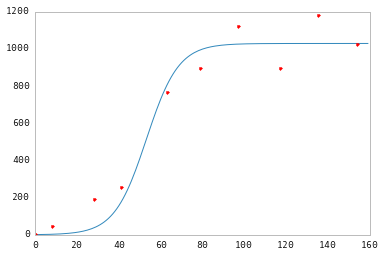### Solution to part 2¶

In :
data = np.c_[days, beetles]
data

Out:
array([[   0,    2],
[   8,   47],
[  28,  192],
[  41,  256],
[  63,  768],
[  79,  896],
[  97, 1120],
[ 117,  896],
[ 135, 1184],
[ 154, 1024]])

Similar to part 1, but with a likelihood such as this:

In :
from scipy.stats.distributions import norm

logistic = lambda t, K, r, N0=1.: K*N0 / (N0 + (K-N0) * np.exp(-r*t))

def likelihood(params, data=data):
K, r, s = params
return -np.sum(norm.logpdf(y, logistic(t, K, r), s) for t,y in data)

In :
from scipy.optimize import minimize

opt_mle = minimize(likelihood, (1000, 0.1, 1), method='Nelder-Mead')

In :
min_func = lambda s: minimize(likelihood, (1000, 0.1, 1), args=s, method='Nelder-Mead')

In :
def bootstrap(data, nsamples, f):
boot_samples = data[np.random.randint(len(data), size=(nsamples, len(data)))]
return [f(s)['x'] for s in boot_samples]

In :
estimates = bootstrap(data, 100, min_func)

In :
np.std(estimates, 0)

Out:
array([  6.57049020e+01,   4.01655756e-02,   1.84341579e+01])
In :
np.corrcoef(np.array(estimates).T[:-1])

Out:
array([[ 1.        , -0.30679739],
[-0.30679739,  1.        ]])
In :
K_opt, r_opt = opt_mle['x'][:-1]

plt.plot(times, logistic(times, K_opt, r_opt))
plt.plot(days, beetles, 'r.')

Out:
[<matplotlib.lines.Line2D at 0x1143b2390>]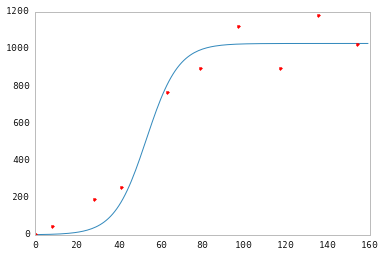## Question 2¶

1. Implement simulated annealing for minimizing the AIC for the baseball salary regression problem. Model your algorithm on the example given in class.

1. Compare the effects of different cooling schedules (different temperatures and different durations at each temperature).
2. Compare the effect of a proposal distribution that is discrete uniform over 2-neighborhoods versus one that is discrete uniform over 3-neighborhoods.
2. Implement a genetic algorithm for minimizing the AIC for the baseball salary regression problem. Model your algorithm on Example 3.5.

1. Compare the effects of using different mutation rates.
2. Compare the effects of using different generation sizes.
3. Instead of the selection mechanism used in the class example, try using independent selection of both parents with probabilities proportional to their fitness.
In :
import pandas as pd

baseball = pd.read_table('../data/textbook/baseball.dat', sep='\s+')

logsalary = baseball.salary.apply(np.log)
predictors = baseball.ix[:, 'average':]
nrows, ncols = predictors.shape

In :
# n * ln(SSE/n) - k * ln(n)
aic = lambda g: g.nobs * np.log((g.resid**2).sum()/g.nobs) + 2*len(g.beta)


This works best if you write a function:

In :
periods = 15
period_lengths = *(periods/3) + *(periods/3) + *(periods/3)
tau_start = 10
cooling_schedule = [tau_start * 0.9**i for i in range(periods)]

def anneal(periods, period_lengths, cooling_schedule, nbrhd=1):

aic_values = []
tau = cooling_schedule

solution_current = solution_best = np.random.binomial(1, 0.5, ncols).astype(bool)
solution_vars = predictors[predictors.columns[solution_current]]
g = pd.ols(y=logsalary, x=solution_vars)
aic_best = aic(g)
aic_values.append(aic_best)

for j in range(periods):

for i in range(period_lengths[j]):

solution_step = solution_current.copy()

flip = np.random.randint(0, ncols, nbrhd)
solution_step[flip] = solution_current[flip] - True
solution_vars = predictors[predictors.columns[solution_step]]
g = pd.ols(y=logsalary, x=solution_vars)
aic_step = aic(g)
alpha = min(1, np.exp((aic_values[-1] - aic_step)/tau[j]))

if ((aic_step < aic_values[-1]) or (random.uniform() < alpha)):
# Accept proposed solution
solution_current = solution_step.copy()
aic_values.append(aic_step)
if aic_step < aic_best:
# Replace previous best with this one
aic_best = aic_step
solution_best = solution_step.copy()
else:
# Retain current solution
aic_values.append(aic_values[-1])

return solution_best, aic_values


### Solution to 1(A)¶

In :
# Schedule 1: cooling = *(periods/3) + *(periods/3) + *(periods/3)
solution1, aic1 = anneal(periods, period_lengths, cooling_schedule)

In :
periods2 = 2000
period_lengths2 = *periods2
cooling_schedule2 = [tau_start * 0.999**i for i in range(periods2)]

solution2, aic2 = anneal(periods2, period_lengths2, cooling_schedule2)

In :
def plot_aic(aic_values, solution_best):
plt.plot(aic_values)
xlim(0, len(aic_values))
xlabel('Iteration'); ylabel('AIC')
aic_best = np.min(aic_values)
print('Best AIC: {0}\nBest solution: {1}\nDiscovered at iteration {2}'.format(aic_best,
np.where(solution_best==True),
np.where(aic_values==aic_best)))
plt.plot(np.where(aic_values==aic_best), aic_best, 'ro')

In :
plot_aic(aic1, solution1)

Best AIC: -416.611888647
Best solution: (array([ 2,  5,  7,  9, 12, 13, 19, 20, 21, 23, 24]),)
Discovered at iteration 1984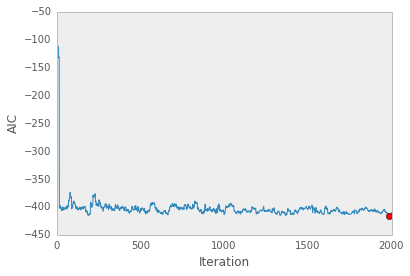In :
plot_aic(aic2, solution2)

Best AIC: -416.087321595
Best solution: (array([ 2,  5,  7,  9, 11, 12, 13, 14, 19, 20, 21]),)
Discovered at iteration 1748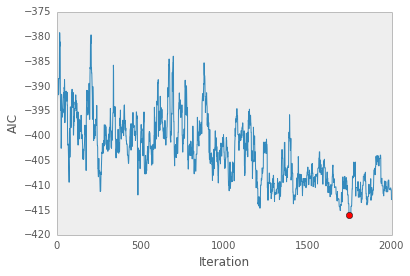### Solution to 1(B)¶

In :
solution_n2, aic_n2 = anneal(periods, period_lengths, cooling_schedule, nbrhd=2)

In :
solution_n3, aic_n3 = anneal(periods, period_lengths, cooling_schedule, nbrhd=3)


### Solution to 2(A)¶

In :
def calculate_fitness(aic_values):
P = len(aic_values)
aic_rank = (-aic_values).argsort().argsort()+1.
return 2.*aic_rank/(P*(P+1.))

In :
def run_ga(pop_size=20, iterations=100, mutation_rate=0.05):

aic_best = []
best_solution = []
aic_history = []

# Initialize genotype
current_gen = np.random.binomial(1, 0.5, pop_size*ncols).reshape((pop_size, ncols))

for i in range(iterations):

# Get phenotype
current_phe = [predictors[predictors.columns[g.astype(bool)]] for g in current_gen]
# Calculate AIC
current_aic = np.array([aic(pd.ols(y=logsalary, x=x)) for x in current_phe])
# Get lowest AIC
aic_best.append(current_aic[np.argmin(current_aic)])
best_solution.append(current_gen[np.argmin(current_aic)])

# Calculate fitness according to AIC rank
fitness = calculate_fitness(current_aic)

# Choose first parents according to fitness
moms = np.random.choice(range(pop_size), size=pop_size/2, p=fitness)
# Choose second parents randomly
dads = np.random.choice(range(pop_size), size=pop_size/2)

next_gen = []
for x,y in zip(current_gen[moms], current_gen[dads]):
# Crossover
cross = np.random.randint(0, ncols)
child1 = np.r_[x[:cross], y[cross:]]
child2 = np.r_[y[:cross], x[cross:]]
# Mutate
m1 = np.random.binomial(1, mutation_rate, size=ncols).astype(bool)
child1[m1] = abs(child1[m1]-1)
m2 = np.random.binomial(1, mutation_rate, size=ncols)
child2[m2] = abs(child1[m2]-1)
next_gen += [child1, child2]

# Increment generation
current_gen = np.array(next_gen)
# Store AIC values
aic_history.append(current_aic)

aic_best_min = np.min(aic_best)

fig, axes = plt.subplots(nrows=1, ncols=2)

print('Best AIC: {0}\nBest solution: {1}\nDiscovered at iteration {2}'.format(aic_best_min,
np.where(best_solution[np.where(aic_history==aic_best_min)]),
np.where(aic_history==aic_best_min)))
axes.plot(aic_best, 'r-')
axes.set_xlim(0, len(aic_best))
axes.set_xlabel('Iteration')
axes.set_ylabel('AIC')

for i,x in enumerate(aic_history):
axes.plot(np.ones(len(x))*i, -x, 'r.', alpha=0.3)

In :
run_ga()

Best AIC: -415.262574256
Best solution: (array([ 0,  2,  5,  6,  7,  9, 12, 13, 14, 15, 21, 23, 24, 25]),)
Discovered at iteration 74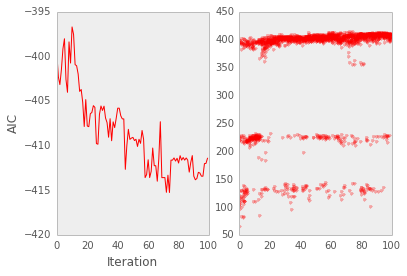In :
run_ga(mutation_rate=0.1)

Best AIC: -414.924853731
Best solution: (array([ 2,  3,  7,  9, 12, 13, 14, 15, 16, 17, 19, 21, 23, 24, 25]),)
Discovered at iteration 60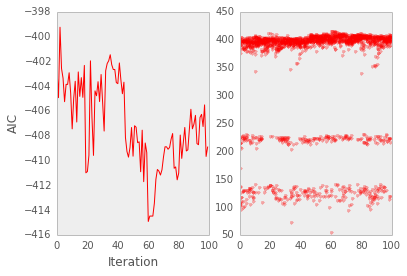In :
run_ga(mutation_rate=0.001)

Best AIC: -408.88454751
Best solution: (array([ 1,  2,  3,  5,  7,  8,  9, 11, 12, 13, 14, 15, 16, 17, 19, 22, 24,
26]),)
Discovered at iteration 40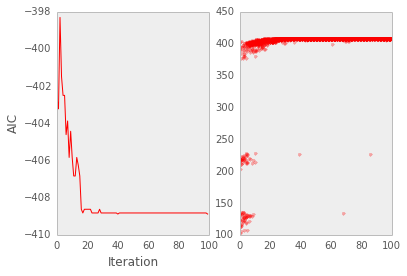### Solution to 2(B)¶

In :
run_ga(pop_size=100, mutation_rate=0.05)

Best AIC: -418.947211437
Best solution: (array([ 1,  2,  5,  7,  9, 12, 13, 14, 15, 23, 24, 25]),)
Discovered at iteration 30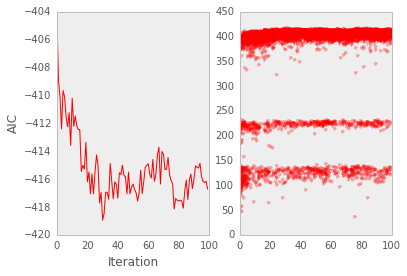In :
run_ga(pop_size=10, mutation_rate=0.05)

Best AIC: -413.821090366
Best solution: (array([ 2,  7,  9, 12, 13, 14, 15, 20, 21, 23, 24, 26]),)
Discovered at iteration 46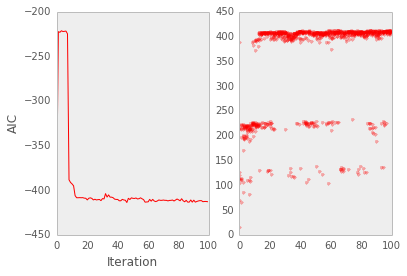### Solution to 2(C)¶

Change selection of dads to include fitness-based probabilities.

In :
def run_ga2(pop_size=20, iterations=100, mutation_rate=0.2):

aic_best = []
best_solution = []
aic_history = []

# Initialize genotype
current_gen = np.random.binomial(1, 0.5, pop_size*ncols).reshape((pop_size, ncols))

for i in range(iterations):

# Get phenotype
current_phe = [predictors[predictors.columns[g.astype(bool)]] for g in current_gen]
# Calculate AIC
current_aic = np.array([aic(pd.ols(y=logsalary, x=x)) for x in current_phe])
# Get lowest AIC
aic_best.append(current_aic[np.argmin(current_aic)])
best_solution.append(current_gen[np.argmin(current_aic)])

# Calculate fitness according to AIC rank
fitness = calculate_fitness(current_aic)

# Choose first parents according to fitness
moms = np.random.choice(range(pop_size), size=pop_size/2, p=fitness)
# Choose second parents randomly
dads = np.random.choice(range(pop_size), size=pop_size/2, p=fitness)

next_gen = []
for x,y in zip(current_gen[moms], current_gen[dads]):
# Crossover
cross = np.random.randint(0, ncols)
child1 = np.r_[x[:cross], y[cross:]]
child2 = np.r_[y[:cross], x[cross:]]
# Mutate
m1 = np.random.binomial(1, mutation_rate, size=ncols).astype(bool)
child1[m1] = abs(child1[m1]-1)
m2 = np.random.binomial(1, mutation_rate, size=ncols)
child2[m2] = abs(child1[m2]-1)
next_gen += [child1, child2]

# Increment generation
current_gen = np.array(next_gen)
# Store AIC values
aic_history.append(current_aic)

aic_best_min = np.min(aic_best)

fig, axes = plt.subplots(nrows=1, ncols=2)

print('Best AIC: {0}\nBest solution: {1}\nDiscovered at iteration {2}'.format(aic_best_min,
np.where(best_solution[np.where(aic_history==aic_best_min)]),
np.where(aic_history==aic_best_min)))
axes.plot(aic_best, 'r-')
axes.set_xlim(0, len(aic_best))
axes.set_xlabel('Iteration')
axes.set_ylabel('AIC')

for i,x in enumerate(aic_history):
axes.plot(np.ones(len(x))*i, -x, 'r.', alpha=0.3)

In :
run_ga2(pop_size=20, mutation_rate=0.05)

Best AIC: -418.947211437
Best solution: (array([ 1,  2,  5,  7,  9, 12, 13, 14, 15, 23, 24, 25]),)
Discovered at iteration 57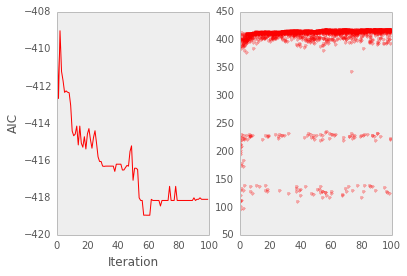## Question 3¶

Use the combinatorial optimization method of your choice to obtain a solution to the traveling salesman problem for the Brazilian cities described in the lecture notes, using minimum total distance as the criterion. Use the the first city listed in the dataset as "home" (i.e. the trip must start and end there. I will award 5 bonus points to the best solution!

In :
def parse_latlon(x):
d, m, s = map(float, x.split(':'))
ms = m/60. + s/3600.
if d<0:
return d - ms
return d + ms

names=['city','lat','lon'])[['lat','lon']].applymap(parse_latlon).values

In :
ncities = len(cities)
periods = 60
cooling = *(periods/3) + *(periods/3) + *(periods/3)
tau_start = 50
tau = [tau_start]*periods
#switches = *(periods/3) + *(periods/3) + *(periods/3)

In :
from scipy.spatial.distance import euclidean

# Calculate total distance among consecutive cities
distance = lambda s: int(np.sum([euclidean(c1,c2) for c1,c2 in zip(cities[s][:-1], cities[s][1:])]))

solution_current = solution_best = np.concatenate([, np.random.permutation(range(1, len(cities))), ])
distance_best = distance(solution_current)
distance_values = [distance_best]

# Cooling schedule
tau = [tau_start * 0.9**i for i in range(periods)]

In :
for j in range(periods):

for i in range(cooling[j]):

solution_step = solution_current.copy()
switch = np.random.randint(1, ncities, 2)
solution_step[switch] = solution_step[switch[::-1]]
d = distance(solution_step)
alpha = min(1, np.exp((distance_values[-1] - d)/tau[j]))
if ((d < distance_values[-1]) or (random.uniform() < alpha)):
# Accept proposed solution
solution_current = solution_step.copy()
distance_values.append(d)
if d < distance_best:
# Replace previous best with this one
distance_best = d
solution_best = solution_step.copy()
else:
# Retain current solution
distance_values.append(distance_values[-1])

In :
plt.plot(distance_values)
xlim(0, len(distance_values))
xlabel('Iteration'); ylabel('Distance')
print('Shortest Distance: {0}\nBest solution: {1}\nDiscovered at iteration {2}'.format(distance_best,
solution_best,
distance_values.index(distance_best)))
plt.plot(distance_values.index(distance_best), distance_best, 'ro')

Shortest Distance: 134
Best solution: [ 0  1  2  3  6  5  7  8  9 11 14 13 16 17 23 25 24 21 19 18 22 20 15 12 10
4  0]
Discovered at iteration 3556

Out:
[<matplotlib.lines.Line2D at 0x1270d44d0>]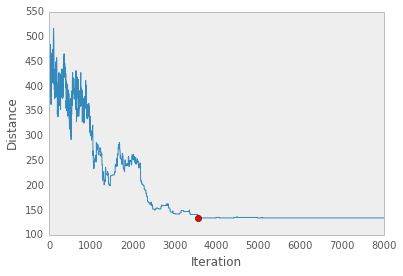In :
from mpl_toolkits.basemap import Basemap

xmin, xmax, ymin, ymax = -70.0, -20.0, -40.0, 10.0

fig = plt.figure(figsize=(11.7,8.3))
bm = Basemap(projection='merc', \
llcrnrlon=xmin, llcrnrlat=ymin, \
urcrnrlon=xmax, urcrnrlat=ymax, \
lon_0=0.5*(xmax + xmin), lat_0=0.5*(ymax + ymin), \
resolution='l', area_thresh=1000000)

bm.drawcoastlines(linewidth=1.5)
bm.bluemarble()
bm.drawcountries(linewidth=2)
bm.drawstates(linewidth=1)

for i,c in enumerate(cities):
pty = 'r.'
if not i:
pty = 'co'
bm.plot(-c, c, pty, latlon=True)
_x, _y = bm(-cities[i], cities[i])
plt.text(_x, _y, repr(int(i)), fontsize=9, color='yellow')

xx, yy = cities[solution_best].T
bm.plot(-xx, yy, 'y--',latlon=True)

Out:
[<matplotlib.lines.Line2D at 0x1333b8050>]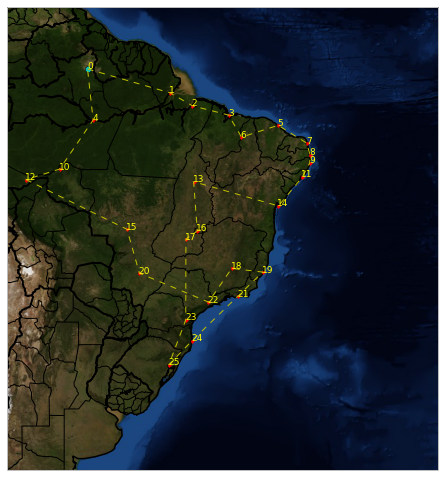## Question 4¶

Suppose $y$ has a binomial distribution with parameters $n$ and $p$, and we are interested in the log-odds value $\theta = \log(p/(1 − p))$. Our prior for $\theta$ is that $\theta \sim N(\mu, \sigma^2)$. It follows that the posterior density of $\theta$ is given, up to a proportionality constant, by:

$$g(\theta | y) \propto \frac{\exp(y\theta)}{(1 + exp(\theta))^n} \exp\left[\frac{-(\theta − \mu)^2}{2\sigma^2}\right]$$

For example, suppose we are interested in learning about the probability that a possibly-biased coin lands heads when tossed. A priori we believe that the coin is fair, so we assign $\theta$ a $N(0,.25)$ prior. We toss the coin $n = 5$ times and obtain $y = 5$ heads.

1. Using a normal approximation to the posterior density, compute the probability that the coin is biased toward heads (i.e., that θ is positive).
2. Using the prior density as a proposal density, design a rejection algorithm for sampling from the posterior distribution. Using simulated draws from your algorithm, approximate the probability that the coin is biased toward heads.
3. Using the prior density as a proposal density, simulate values from the posterior distribution using the SIR algorithm. Approximate the probability that the coin is biased toward heads.

### Solution to Part 1¶

In :
def logodds_post(theta, n=5, y=5, mu=0, sigma2=0.25):

lpost = y*theta - n*np.log(1. + np.exp(theta))
lpost -= (theta - mu)**2 / (2*sigma2)

return lpost

In :
logodds_post_min = lambda *args: -logodds_post(*args)

In :
from scipy.optimize import fmin_bfgs

opt = fmin_bfgs(logodds_post_min, 1., full_output=True)
mode, var = opt, opt
mode, var

Optimization terminated successfully.
Current function value: 2.869167
Iterations: 3
Function evaluations: 15

Out:
(array([ 0.47831325]), array([[ 0.19307885]]))

Probability that coin is biased:

In :
from scipy.stats.distributions import norm

1. - norm.cdf(0, loc=mode, scale=np.sqrt(var))

Out:
0.8618219756399822

### Solution to Part 2¶

First need to calculate scaling constant C:

In :
def calc_diff(theta):

return logodds_post(theta) - norm.logpdf(theta, loc=0, scale=0.25)

calc_diff_min = lambda *args: -calc_diff(*args)

In :
opt = fmin_bfgs(calc_diff_min,
1,
full_output=True)

Warning: Desired error not necessarily achieved due to precision loss.
Current function value: -3.966336
Iterations: 0
Function evaluations: 57

In :
c = opt
c

Out:
array([-3.96633573])
In :
def reject(n, c, mu=0, s=0.25):

k = len(mode)

# Draw samples from g(theta)
theta = norm.rvs(loc=mu, scale=s, size=n)

# Calculate probability under g(theta)
gvals = norm.logpdf(theta, loc=mu, scale=np.sqrt(s))

# Calculate probability under f(theta)
fvals = np.array([logodds_post(t) for t in theta])

# Calculate acceptance probability
p = np.exp(fvals - gvals + c)

return theta[np.random.random(n) < p]

In :
nsamples = 1000000
samples = reject(nsamples, c)

In :
np.mean(samples>0)

Out:
0.72345679012345676

### Solution to Part 3¶

In :
n = 100000
mu, s = mode, var
theta = norm.rvs(loc=mu, scale=np.sqrt(s), size=n)

In :
f_theta = np.array([logodds_post(t) for t in theta])

In :
q_theta = norm.logpdf(theta, loc=mu, scale=np.sqrt(s))


Importance weights

In :
w = np.exp(f_theta - q_theta - max(f_theta - q_theta))

In :
p_sir = w/w.sum()

In :
theta_sir = theta[np.random.choice(range(len(theta)), size=10000, p=p_sir)]

In :
plt.hist(theta_sir)

Out:
(array([    6.,    32.,   314.,  1304.,  2769.,  3037.,  1818.,   595.,
115.,    10.]),
array([-1.40089395, -1.03821797, -0.675542  , -0.31286602,  0.04980996,
0.41248593,  0.77516191,  1.13783788,  1.50051386,  1.86318984,
2.22586581]),
<a list of 10 Patch objects>)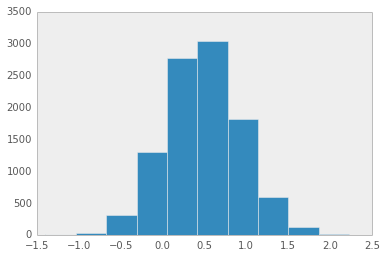In :
np.mean(theta_sir>0)

Out:
0.86140000000000005